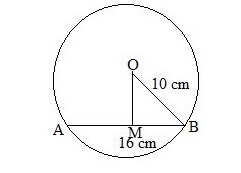# A chord of length 16 cm is drawn in a circle of radius 10 cm.

Question:

A chord of length 16 cm is drawn in a circle of radius 10 cm. Find the distance of the chord from the centre of the circle.

Solution:

Let AB be the chord of the given circle with centre O and a radius of 10 cm.
Then AB =16 cm and OB = 10 cmFrom O, draw OM perpendicular to AB.
We know that the perpendicular from the centre of a circle to a chord bisects the chord.

$\therefore B M=\left(\frac{16}{2}\right) \mathrm{cm}=8 \mathrm{~cm}$

In the right  ΔOMB, we have:
OB2 = OM2 + MB2   (Pythagoras theorem)
⇒ 102 = OM2 + 82
⇒ 100 = OM2 + 64
⇒ OM2 = (100 - 64) = 36

$\Rightarrow O M=\sqrt{36} \mathrm{~cm}=6 \mathrm{~cm}$

Hence, the distance of the chord from the centre is 6 cm.